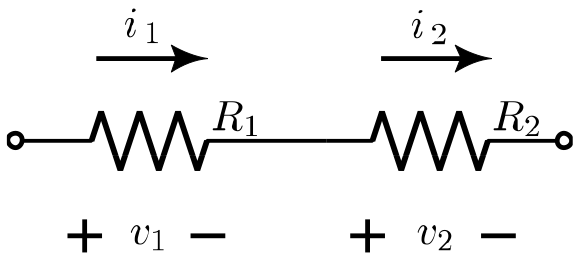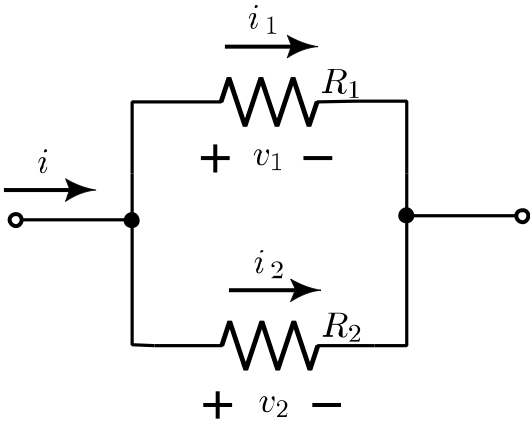“Resistance is useless.” – Daleks; Doctor Who

Resistors in series: $$R_{1} + R_{2} + R_{3}$$

Resistors in parallel: $$\left ( \frac{1}{R_{1}} + \frac{1}{R_{2}} + \frac{1}{R_{3}} \right )^{-1}$$

Voltage divider (using resistors in series): $$v_1=\frac{R_1}{R_1+R_2}v$$• $$v_1$$ = The voltage across the first resistor
• v = The voltage across both resistors

Current divider (using resistors in parallel): $$i_1=\frac{R_2}{R_1+R_2}i$$• $$i_1$$ = The current through the first resistor.

At RF, all resistors act like a resistor, an inductor, and a capacitor. For the three major types of resistors:

• Carbon composition resistors - act a lot like a capacitor, due to the capacitance between the granules.
• Wirewound resistors - act a lot like an inductor, because the wire looks a lot like a coil.
• Metal film resistors - act closer to an ideal resistor (but not perfectly so) than the other types. “Thin film” surface mount are the best of the best.